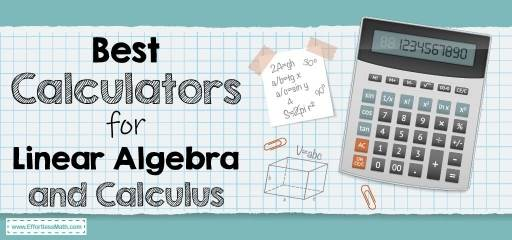# Best Calculators for Linear Algebra and CalculusLinear algebra and calculus are some of the most technical math courses. Solving without any aid could be difficult as well as time-consuming.

With the use of the right calculator, you will be able to optimize your calculations. Not all calculators can be used for linear Algebra and Calculus.

In this article, we will be discussing some of the best calculators for linear algebra and calculus.

The Absolute Best Book for the Algebra 1

## Four Best Calculators for Linear Algebra

In linear Algebra, the main calculations include calculating the determinant of the matrices as well as the inverse. Calculators for linear algebra must be able to; Calculate eigenvectors and eigenvalues. And carry out RREF (Reduce row echelon form).

Since matrix operations are always time-consuming, these features are shortcuts to getting accurate answers.

Listed below are the best calculators for linear Algebra and Calculus.

### TI-84 Plus CE Color Graphing Calculator (Black)

This is one of the best calculators made by Texas Instruments. Just like a smartphone, you need to download free apps which will expand its capability in solving linear algebra equations.

It can be used for intensive computational modules such as computational physics among others. Guess what? It’s a complete calculator as can be used both at undergraduate and graduate levels.

Features

• It is used in solving simultaneous equations
• For calculating Eigenvalues, LDU composition, and Cholesky Factorization.
• It is used in performing Gram Schmidt Orthonormalization.
• For calculating the adjoint of a matrix

### TI-84 Plus Graphing Calculator

The Texas 84 Plus Graphing Calculator is a black-and-white version of the TI-84 Plus CE Color Graphing Calculator. It is budget-friendly

Features

### TI-83 Plus Graphing Calculator

It is the older version and yet backs up the linear Algebra apps.

Features

• It is cheaper
• It can be used for other linear algebra courses such as differential equations.
• It performs Gram Schmidt Orthonormalization.

### TI-89 Titanium Graphing Calculator

They are the newer fashion with an inbuilt Matrix mode. This made it possible to carry out basic matrix operations.

Features

• Performs basic matrix operations like the inverse.
• They are used for LU decomposition
• Used in finding Eigenvalues and Eigenvectors

The Absolute Best Book to Ace the Algebra 2 Test

## Best Calculators for Calculus

In choosing a calculator for calculus, ensure it can carry out numerical differentiation and integration. It should also be able to find the derivative of a function. Below is a list of the best calculators for calculus.

### TI-Nspire CX II CAS Color Graphing Calculator

Computer Algebra System (CAS) depicts that the calculator can give both symbolic and numerical results. It is a smart calculator with student software.

Features

• It consists of a dissolve wizard function which reduces syntax errors in solving differential equations.
• Can perform vector calculus

### TI-84 Plus CE Color Graphing Calculator (White)

It carries out symbolic differentiation which involves finding the derivative of both symbol and also numerical value at certain values of x.

### Casio fx-9750 GII Graphing Calculator

It is white with an icon-based menu. It performs operations such as calculations of determinants of the matrix, inverse, and also the multiplication of matrices.

Casio calculators are low in price compared to the TI series but lack some features which can be found in the TI series.

### Hp 50g Graphing Calculator

It can be used for performing a wide range of calculus. This is because it is like an Excel spreadsheet.

After inputting the matrix, you can perform operations such as transpose, trace, finding the determinant and rank of a matrix.

It is an advanced fashion of calculator for calculus.

Features

• It can perform multiple integrals
• For calculating the Fourier series
• To Calculate the Taylor/Maclaurin series of a function symbolically (up to 4th lack relative power, i.e., the difference between the highest and lowest power in the expansion is 4)
• It can be used for antiderivatives
• Performs vector calculus such as del, gradient, curl, and divergence

## Conclusion

By now, you already know the best calculators for linear Algebra and the best calculators for calculus. Go ahead and choose which you’d like to get.

The Best Books to Ace the Algebra 2

### What people say about "Best Calculators for Linear Algebra and Calculus - Effortless Math: We Help Students Learn to LOVE Mathematics"?

No one replied yet.

X
28% OFF

Limited time only!

Save Over 28%

SAVE \$5

It was \$17.99 now it is \$12.99